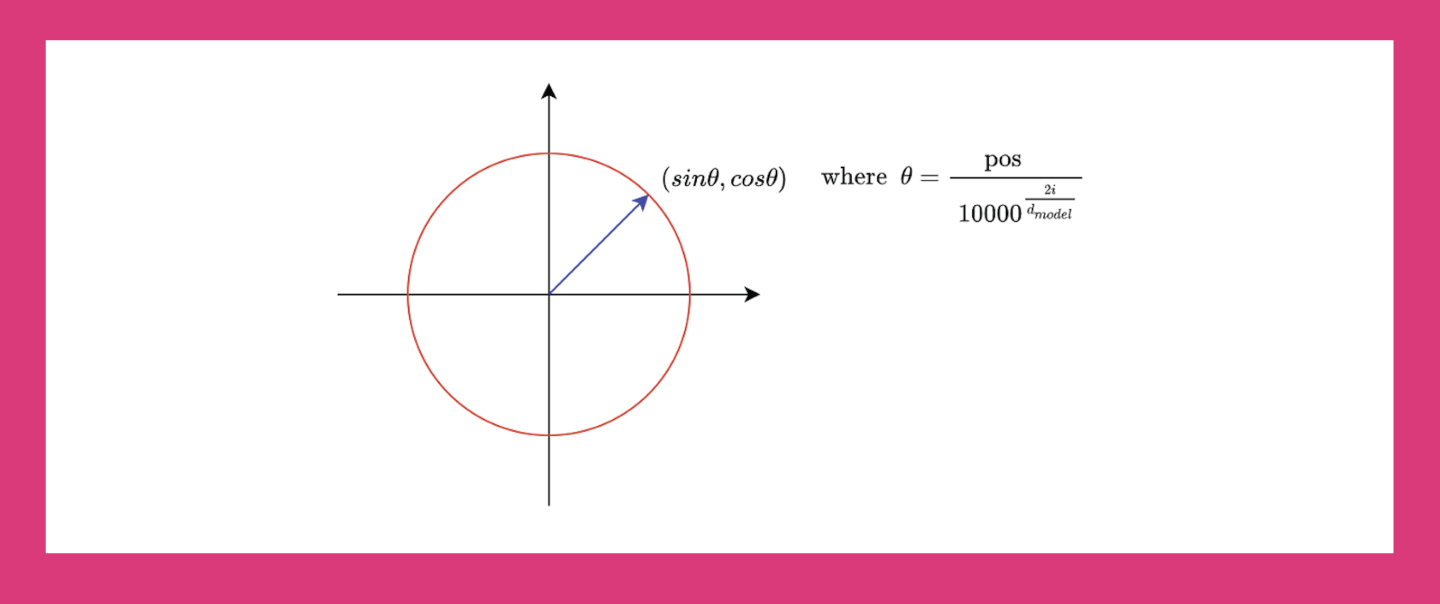# What is Positional Encoding

Embed the positions of entities in a sequence.

Good knowledge of Neural networks, embeddings, and LSTM networks.

# Why do we need Positional encoding

Classical neural network models do not account for sequence information.

Up to now for using the sequence information we have used LSTM cells which have positional information but the problem with them is they cannot be parallelized, and you must feed one vector at a time, so they are slow to train.

The Transformers which use positional encoding are built on the structure of the classical neural networks and use positional encoding to get the sequence information.

First, let’s see how the data is fed to a transformer: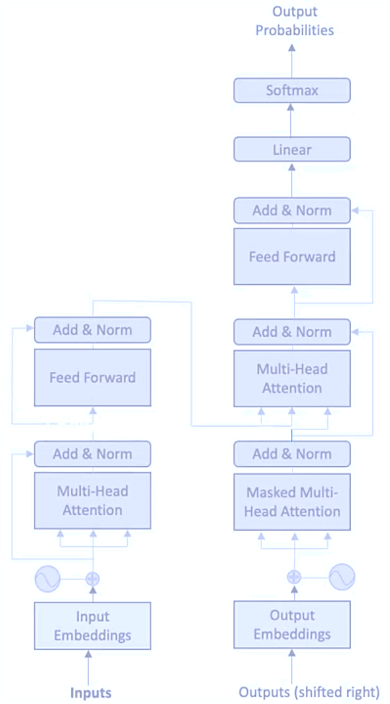So, there are two different things involved before Positional encoding:

• Inputs and,

• Input embedding

## Inputs and Input Embeddings

First, we have a sentence we convert into a vector.

Input is our sentence, which is to be converted into a vector then we convert this vector into an embedding vector that is fed to the positional encoding part.

Example:

Take an input Text –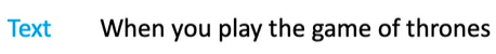Now convert this to a vector with the help of vocabulary, where we take indices of vocabulary for the input text.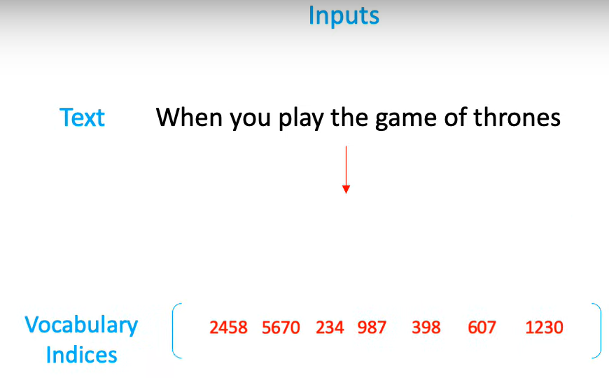Now we need to convert this vector into an embedding, for this, we need a trained embedding layer, which has a vector representation for every word in our dictionary.

What and how do we get an embedding file/ layer? In an embedding file, we have an index for a word and a vector (say 300 dimensions) attached to it, which first has random values but after training it gets changed depending upon relation with other words in a sentence (in simple words we can say depending upon the meaning of the word).

So, after embedding the layer we get something like this: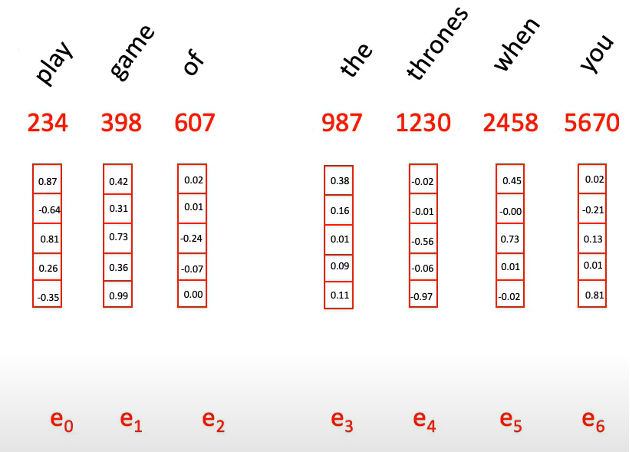This is just an illustration of an embedding vector, in real embeddings vector mainly has a size of more than 100.

## Positional Encoding

Now we need to feed these vectors to the Transformers but before doing this we need to attach some number to this vector that can tell the Transformer how the sequence of these vectors looks in a sentence. As sequence information is a lot useful in NLP tasks. So, for doing that we have positional encoding, where they use wave frequency to capture the positional information. Formulas look like this: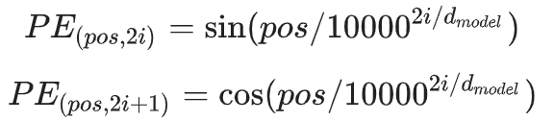But why not just use a whole number or number between 0 to 1? To get position information.

Say we use positive whole numbers and attach that to the embedding like this: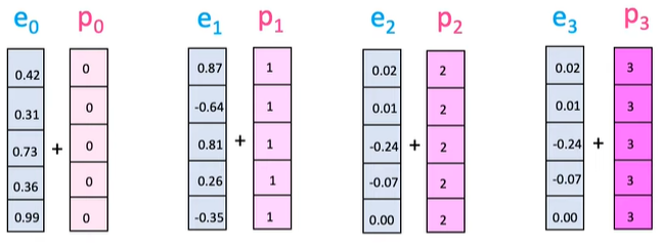The problem with this is that the large values for the whole number hugely distort the embedding values to the word embedding that comes later in the sentence. Consider the whole number 30 being added to the word embedding.

Now let’s try only with values between 0 to 1: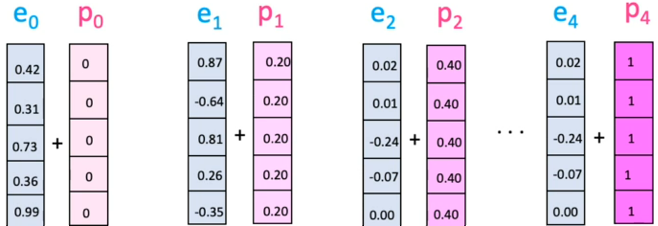So, we get these number that is between 0 to 1. Depending on the length of the number like: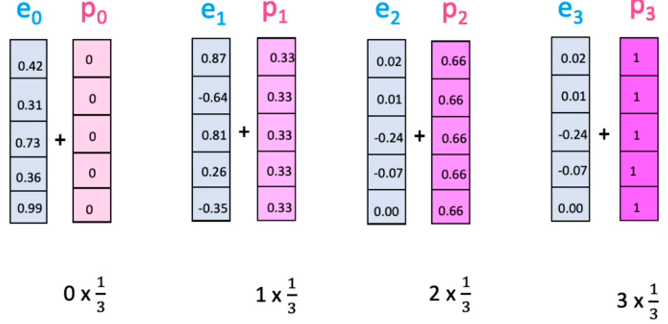As we are getting these numbers depending on the length of the sentence. The value of this number will vary with a change in sentence length. So, you would be adding a different number to the same position with a change in sentence length.

So the people from transformer paper came up with a clever idea of using sin and cos wave frequency to encode the positional information.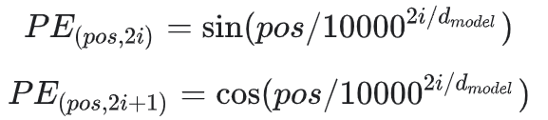They apply the sin formula to even position words and cos to odd position words.

Here “pos” refers to the position of the “word” in the sequence. P0 refers to the position embedding of the first word; “d” means the size of the word/token embedding. In this example d=5. Finally, “i” refers to each of the 5 individual dimensions of the embedding (i.e., 0, 1,2,3,4)

While “d” is fixed, “pos” and “i” vary. Let us try understanding the latter two.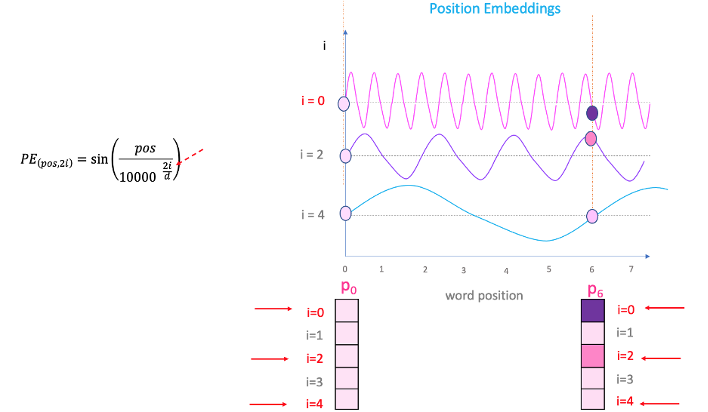If we plot a sin curve and vary “pos” (on the x-axis), you will land up with different position values on the y-axis. Therefore, words with different positions will have different position embedding values.

There is a problem though. Since “sin” curve repeat in intervals, you can see in the figure above that P0 and P6 have the same position embedding values, despite being at two very different positions. This is where the ‘i’ part in the equation comes into play.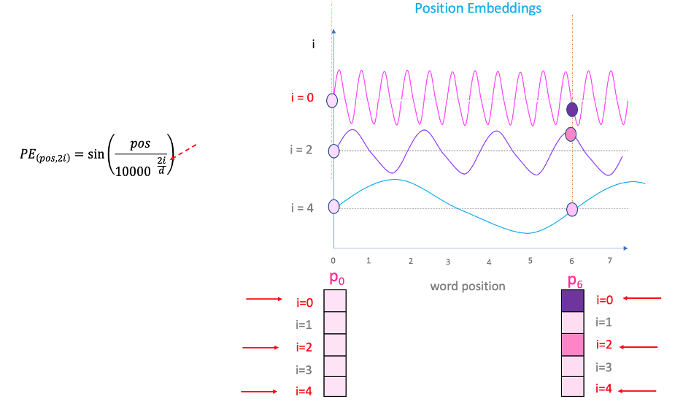If you vary “i” in the equation above, you will get a bunch of curves with varying frequencies. Reading off the position embedding values against different frequencies, lands up giving different values at different embedding dimensions for P0 and P6.

We have reviewed the basic knowledge and approach of positional encoding. We will review and implement a case of positional encoding with code example in our next article.